# How to extract number from string in Excel

The tutorial shows how to extract number from various text strings in Excel by using formulas and the Extract tool.

When it comes to extracting part of a text string of a given length, Excel provides three Substring functions (Left, Right and Mid) to quickly handle the task. When it comes to extracting numbers from an alphanumeric string, Microsoft Excel provides… nothing.

To get a number from a string in Excel, it takes a little ingenuity, a bit of patience, and a bunch of different functions nested into each other. Or, you can run the Extract tool and have the job done with a mouse click. Below you will find full details on both methods.

## How to extract number from the end of text string

When you have a column of alphanumeric strings where number comes after text, you can use the following formula to get it.

RIGHT(cell, LEN(cell) - MAX(IF(ISNUMBER(MID(cell, ROW(INDIRECT("1:"&LEN(cell))), 1) *1)=FALSE, ROW(INDIRECT("1:"&LEN(cell))), 0)))

We will dwell on the formula's logic a bit later. For now, simply replace cell with a reference to the cell containing the original string (A2 in our case), and enter the formula in any empty cell in the same row, say in B2:

`=RIGHT(A2, LEN(A2) - MAX(IF(ISNUMBER(MID(A2, ROW(INDIRECT("1:"&LEN(A2))), 1) *1)=FALSE, ROW(INDIRECT("1:"&LEN(A2))), 0)))`

This formula gets number only from the end. If a string also has numbers in the beginning or middle, they are ignored: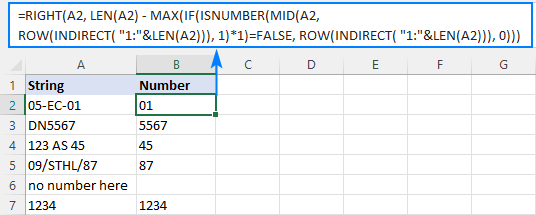The extraction is performed with the RIGHT function that belongs to the category of Text functions. The output of this function is always text. In our case, the result is a numeric substring, which in terms of Excel is also text, not number.

If you need the result to be a number (that you can use in further calculations), then wrap the formula into the VALUE function or perform an arithmetic operation that does not change the result, say, multiply by 1 or add 0. To catch errors in the strings that do not contain a single number, use the IFERROR function. For example:

`=IFERROR(VALUE(RIGHT(A2, LEN(A2) - MAX(IF(ISNUMBER(MID(A2, ROW(INDIRECT("1:"&LEN(A2))), 1)*1)=FALSE, ROW(INDIRECT("1:"&LEN(A2))), 0)))), "")`

or

`=IFERROR(RIGHT(A2, LEN(A2) - MAX(IF(ISNUMBER(MID(A2, ROW(INDIRECT("1:"&LEN(A2))), 1) *1)=FALSE, ROW(INDIRECT("1:"&LEN(A2))), 0))) +0, "")`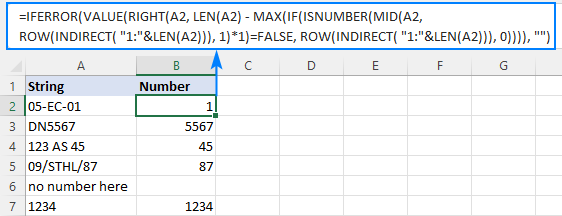Note. In Dynamic Array Excel (Office 365 and 2021), you enter the formula in the usual way with the Enter key. In Excel 2019 and earlier, it only works as an array formula, so remember to press Ctrl + Shift + Enter to complete it.

How this formula works:

To extract number from an alphanumeric string, the first thing you need to know is where to start the extraction. The position of the last non-numeric character in a string is determined with the help of this tricky formula:

MAX(IF(ISNUMBER(MID(A2, ROW(INDIRECT("1:"&LEN(A2))), 1)*1)=FALSE, ROW(INDIRECT("1:"&LEN(A2))), 0))

To understand the logic, let's investigate it from the inside:

The ROW(INDIRECT("1:"&LEN(A2))) combination creates a sequence of numbers corresponding to the total of characters in the source string (A2), and we serve these sequential numbers to MID as the starting numbers:

MID(A2, {1;2;3;4;5;6;7;8}, 1)

The MID function pulls each individual character from A2 and returns them as an array:

{"0";"5";"-";"E";"C";"-";"0";"1"}

As MID is a text function, its output is always text (as you can notice, all the characters are enclosed in quotation marks). To turn numeric ones into numbers, we multiply the array by 1 (double negation --MID() will have the same effect). The result of this operation is an array of numbers and #VALUE! errors representing non-numeric characters:

ISNUMBER({0;5;#VALUE!;#VALUE!;#VALUE!;#VALUE!;0;1})

The ISNUMBER function evaluates each element of the array and gives its verdict in the form of Boolean values - TRUE for numbers, FALSE for anything else:

{TRUE;TRUE;FALSE;FALSE;FALSE;FALSE;TRUE;TRUE}

This array goes to the logical test of the IF function, where each element of the array is compared against FALSE:

IF({TRUE;TRUE;FALSE;FALSE;FALSE;FALSE;TRUE;TRUE}=FALSE, ROW(INDIRECT("1:"&LEN(A2))), 0)

For each FALSE (non-numeric value), another ROW(INDIRECT()) function returns its relative position in the string. For each TRUE (numeric value), a zero is returned. The resulting array looks as follows:

{0;0;3;4;5;6;0;0}

The rest is easy. The MAX function finds the highest number in the above array, which is the position of the last non-numeric value in the string (6 in our case). Simply, subtract that position from the total length of the string returned by LEN, and pass the result to RIGHT to let it know how many characters to extract from the right side of the string:

RIGHT(A2, LEN(A2) - 6)

Done!

## How to extract number from the beginning of text string

If you are working with records where text appears after number, you can extract number from the start of a string by using this generic formula:

LEFT(cell, MATCH(FALSE, ISNUMBER(MID(cell, ROW(INDIRECT("1:"&LEN(cell)+1)), 1) *1), 0) -1)

With the original string in A2, use the following formula to get number:

`=LEFT(A2, MATCH(FALSE, ISNUMBER(MID(A2, ROW(INDIRECT("1:"&LEN(A2)+1)), 1) *1), 0) -1)`

No matter how many digits are in the middle or end, only the starting number is extracted: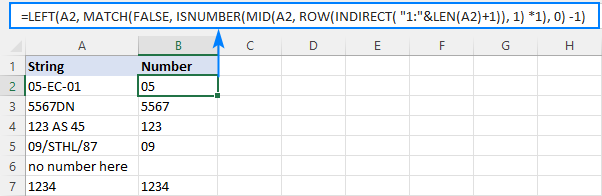Note. In Excel 365 and Excel 2021, due to support for dynamic arrays, a regular formula works fine. In Excel 2019 and earlier, you should press Ctrl + Shift + Enter to explicitly make it an array formula.

How this formula works:

Here, we again use the combination of ROW, INDIRECT and LEN functions to create a sequence of numbers equal to the total of characters in the source string plus 1 (the role of that additional character will become clear a bit later).

ROW(INDIRECT("1:"&LEN(A2)+1))

MID and ISNUMBER do the same job as in the previous example - MID pulls individual characters and ISNUMBER converts them to the logical values. The resulting array of TRUE's and FALSE's goes to the MATCH function as a lookup array:

MATCH(FALSE, {TRUE;TRUE;FALSE;FALSE;FALSE;FALSE;TRUE;TRUE;FALSE}, 0)

MATCH computes a relative position of the first FALSE, giving us the position of the first non-numeric character in the string (3 in A2). To extract the preceding numbers, we subtract 1 from position the first text character and serve the difference to the num_chars argument of the LEFT function:

LEFT(A2, 3-1)

Now, back to an "extra" character in the sequence generated by ROW(INDIRECT()+1)). As you already know, this sequence provides the starting points for the MID function. Without +1, MID would extract exactly as many characters as there are in the original string. If the string contains only numbers, ISNUMBER will return only TRUE's while MATCH needs at least one FALSE. To ensure that, we add one more character to the total length of the string, which the MID function would convert to an empty string. For example, in B7, MID returns this array:

{"1";"2";"3";"4";""}

Note. As is the case with the RIGHT function, LEFT also returns a numeric substring, which is technically text, not number. To get the result as a number rather than a numeric string, nest the formula in the VALUE function or multiply the result by 1 as shown in the first example.

## How to get number from any position in a string

If your task implies extracting number from anywhere in a string, you can make use of the following mind-boggling formula published on MrExcel forum:

`=SUMPRODUCT(MID(0&A2, LARGE(INDEX(ISNUMBER(--MID(A2, ROW(INDIRECT("1:"&LEN(A2))), 1)) * ROW(INDIRECT("1:"&LEN(A2))), 0), ROW(INDIRECT("1:"&LEN(A2))))+1, 1) * 10^ROW(INDIRECT("1:"&LEN(A2)))/10)`

Where A2 is the original text string.

Breaking down this formula would require a separate article, so you can simply copy it to your worksheet to make sure it really works :)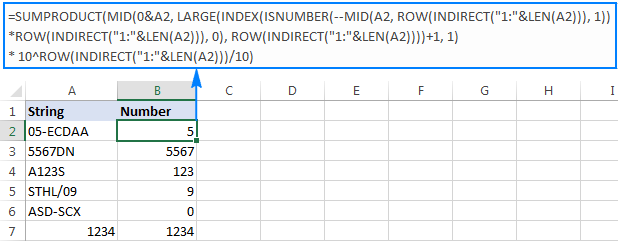Upon examining the results, however, you may notice one insignificant drawback - if the source string does not contain a number, the formula returns zero, as in row 6 in the screenshot above. To fix this, you can wrap the formula in the IF statement, the logical test of which checks if the source string contains any number. If it does, the formula extracts the number, otherwise returns an empty string:

`=IF(SUM(LEN(A2)-LEN(SUBSTITUTE(A2, {"0","1","2","3","4","5","6","7","8","9"}, "")))>0, SUMPRODUCT(MID(0&A2, LARGE(INDEX(ISNUMBER(--MID(A2,ROW(INDIRECT("\$1:\$"&LEN(A2))),1))* ROW(INDIRECT("\$1:\$"&LEN(A2))),0), ROW(INDIRECT("\$1:\$"&LEN(A2))))+1,1) * 10^ROW(INDIRECT("\$1:\$"&LEN(A2)))/10),"")`

As shown in the screenshot below, the improved formula works beautifully (kudos to Alex, our Excel guru, for this improvement):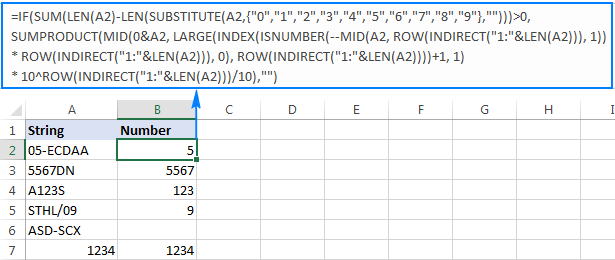Unlike in all previous examples, the result of this formula is number. To make sure of this, just notice the right-aligned values in column B and truncated leading zeros.

Tip. In Excel 365 - Excel 2019, there is a much simpler solution with the help of the TEXTJOIN function. Please see How to remove text and keep numbers.

## Extract number from text string with Ultimate Suite

As you have just seen, there is no trivial Excel formula to pull number from a text string. If you have difficulties with understanding the formulas or tweaking them for your data sets, you may like this simple way to get number from string in Excel.

With our Ultimate Suite added to your Excel ribbon, this is how you can quickly retrieve number from any alphanumeric string:

1. Go to the Ablebits Data tab > Text group, and click Extract: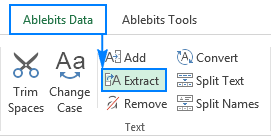2. Select all cells with the source strings.
3. On the Extract tool's pane, select the Extract numbers radio button.
4. Depending on whether you want the results to be formulas or values, select the Insert as formula box or leave it unselected (default).

My advice is to select this box if you want the extracted numbers to update automatically as soon as any changes are made to the source strings. If you want the results to be independent on the original strings (e.g. in case you plan to remove the source data at a later point), then do not select this box.

5. Click the Insert Results button. Done!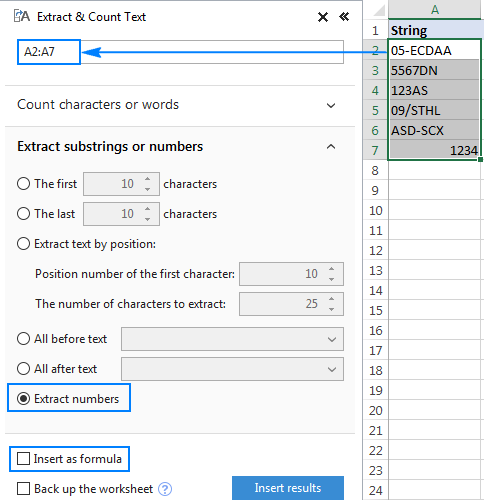Like in the previous example, the results of the extraction are numbers, meaning you are free to count, sum, average, or perform any other calculations with them.

In this example, we've chosen to insert the results as values, and the add-in did exactly what was asked for: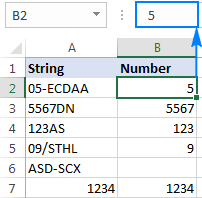If the Insert as formula checkbox was selected, you'd observe a formula in the formula bar. Curious to know which one? Just download Ultimate Suite's trial and see for yourself :)

Excel Extract Number - sample workbook (.xlsx file)
Ultimate Suite - trial version (.exe file)